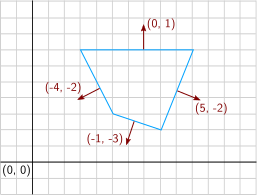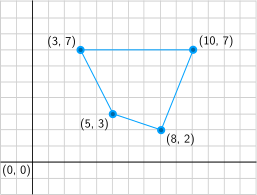# Polyhedra and polytopes

Polyhedra are geometric objects that appear in mechanics to represent power constraints such as friction cones and maximum torque limits.

## Representations

A subset $$P \subset \mathbb{R}^d$$ is called a (convex) polyhedron when it is the set of solutions to a finite system of linear inequalities. In matrix form:

\begin{equation*} P = \{x \ | \ A x \leq b\} \end{equation*}

where $$A$$ is an $$n \times d$$ matrix and $$b$$ is a $$d$$-dimensional vector (vector inequalities are componentwise). When a polyhedron is bounded, it is called a polytope. To mark the difference between 2D and higher dimensions, 2-dimensional polytopes are called polygons.

### Halfspace representation

The halfspace representation (H-rep for short) is the description of the polyhedron by inequalities:

\begin{equation*} A x \leq b \end{equation*}

It is always possible to normalize the representation so that the components of $$b$$ are either 1 or 0. When all these components are zero, the polyhedron is a polyhedral convex cone pointed at the origin. When all the components of $$b$$ are ones, nothing can be said for sure... But, the other way round, a polytope has only ones in the column vector of its H-rep. We therefore end up with the following vocabulary:

\begin{equation*} \begin{array}{rl} \text{Polyhedron:} & P = \{ x \ | \ A x \leq b\} \\ \text{Polyhedral cone:} & P = \{ x \ | \ A x \leq 0\} \\ \text{Polytope:} & P = \{ x \ | \ A x \leq 1\} \end{array} \end{equation*}In the 2D example to the right, the blue polygon is defined by four halfplanes. Here is a full H-rep of this polygon:

\begin{equation*} \begin{bmatrix} 0 & 1 \\ 5 & -2 \\ -1 & -3 \\ -4 & -2 \end{bmatrix} \begin{bmatrix} x \\ y \end{bmatrix} \leq \begin{bmatrix} 7 \\ 36 \\ -14 \\ -26 \end{bmatrix} \end{equation*}

Normals corresponding to each supporting halfplane are depicted by red arrows (note that the length of the arrows is normalized on this drawing and does not correspond to that of the coordinates written next to it). Each line of the matrix $$A$$ corresponds to one normal, while the corresponding column in $$b$$ represents the affine offset between the halfplane and the origin.

### Vertex representation

The vertex representation (V-rep for short) of a polyhedron describes it in terms of points (its vertices) and generating vectors called rays. Informally, vertices are the "finite" boundaries of the polyhedron, while rays are the "infinite" ones. A polytope has only vertices, while a polyhedral cone has only rays. Formally, points $$x$$ of the polyhedron are described by:

\begin{equation*} x = \mathrm{conv}(V) + \mathrm{coni}(R) \end{equation*}

where $$\mathrm{conv}$$ denotes the convex hull of a set of vertices $$V = \{ v_1, \ldots, v_p \}$$:

\begin{equation*} \mathrm{conv}(V) = \left\{ \sum_{i=1}^p \alpha_i v_i \ \textrm{ s.t. } \ \forall i, \alpha_i \geq 0, \sum_{i=1}^p \alpha_i = 1 \right\} \end{equation*}

while $$\mathrm{coni}$$ is the conical hull of a set of rays $$R = \{ r_1, \ldots, v_q\}$$:

\begin{equation*} \mathrm{coni}(R) = \left\{ \sum_{j=1}^q \lambda_j r_j \ \textrm{ s.t. } \ \forall j, \lambda_j \geq 0\right\} \end{equation*}In our 2D example to the right, the polyhedron is a polytope, so that $$R = \emptyset$$. The four vertices of its V-rep are given by

\begin{equation*} V = \{(3, 7), (10, 7), (8, 2), (5, 3)\} \end{equation*}

In this particular case, the representation is unique, contrary to halfspace representations where each line can be multiplied by a positive constant without changing the underlying polyhedron. This uniqueness is basically only true for polytopes. In the general case where $$R \neq \emptyset$$, rays can be scaled as well by positive constants without changing the underlying polyhedron.

## Double description

So far, we formally defined polyhedra from their H-rep, and introduced the V-rep. A fundamental result in polyhedral geometry states that these two representations are interchangeable:

Theorem (Minkowski and Weyl): any polyhedron can be equivalently described in halfspace or vertex representation. That is, for any set $$P = \{ x \ | \ A x \leq b\}$$, there exists two sets $$V$$ and $$R$$ such that $$P = \mathrm{conv}(V) + \mathrm{coni}(R)$$; and conversely.

From a computational standpoint, however, these two representations are not exactly equivalent. For example:

• With the halfspace representation, it is easy to check whether a point $$x$$ belongs to the polytope $$(x \in P)$$, but hard to sample points inside $$P$$.
• With the vertex representation, it is hard to check whether $$x \in P$$ (this amounts to solving a linear program) but easy to sample points inside $$P$$ (using random values for the coefficients $$\alpha_i$$ and $$\lambda_j$$ in the convex and conic hulls, respectively).

Converting from a vertex to a halfspace representation is known as the facet enumeration problem, while conversely converting from a halfspace to a vertex representation is the vertex enumeration problem. These two problems are actually equivalent when put under an appropriate mathematical formulation, and can be solved using the double description method. The cdd library provides a C implementation of this algorithm.